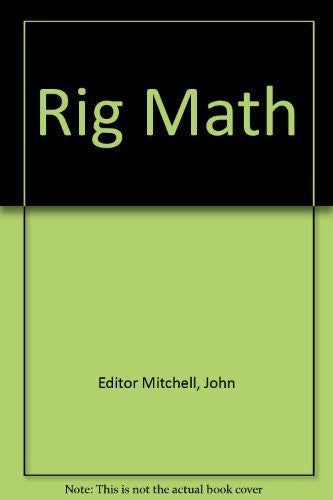# Rig Math

: brown-tech

: 1110000021626

: Book

\$142.50

#### Details

Chapter 1 The Need for Math Skills
Chapter 2 Basic Arithmetic
Mathematical Operations
The Hierarchy of Operations in Equations
Exercise 1
Exercise 2
Exercise 3
Exercise 4
Exercise 5
Chapter 3 Scientific Calculators
Exercise 6
Chapter 4 Units of Measurement
Units of Measurement
Measurements
Time
Money
Depth
Length, Height, and Width
Area
Volume
Pressure
Density
Weight
Percentages
Torque
Distance, Velocity, and Acceleration
Summary
Exercise 7
Exercise 8
Chapter 5 Fractions
Multiplication of Fractions
Division of Fractions
Fractions with Words
Decimal equivalents
Exercise 9:
Chapter 6 Conversion Factors
Length
Weight
Volume
Density
Exercise 10:
Exercise 11:
Chapter 7 Elementary Algebra
Equations with Variables
Solving for Unknown Variables.
Exercise 12:
Chapter 8 Practical Problem Solving – Well Control
Kill Weight Mud
Pumping Kill Weight Mud to the Bit
Initial Circulating Pressure
Final Circulating Pressure
Calculating Strokes to Bit
Preparing the Pumping Schedule
Exercise 13:
Exercise 14:
Exercise 15:
Exercise 16:
Chapter 9 Practical Problem Solving – Lost Circulation
Exercise 17
Chapter 10 Practical Problem Solving – Slugging the Pipe
Managing Hole Fill While Tripping
Chapter 11 Homework for Practical Problem Solving
Exercise 19 Pressure (1 set 5 reps)
Exercise 20 Displacement (1 set 7 reps)
Exercise 21 Buoyancy (1 set 4 reps)
Exercise 22 Torque
Answers to Exercise 18 - 22
Volume(1 set 7 reps)
Pressure(1 set 5 reps)
Displacement(1 set 7 reps)
Buoyancy(1 set 4 reps)
Torque(1 set 3 reps)
Appendix A Conversion Factors
Appendix B Volume of fluid in a horizontal tank.